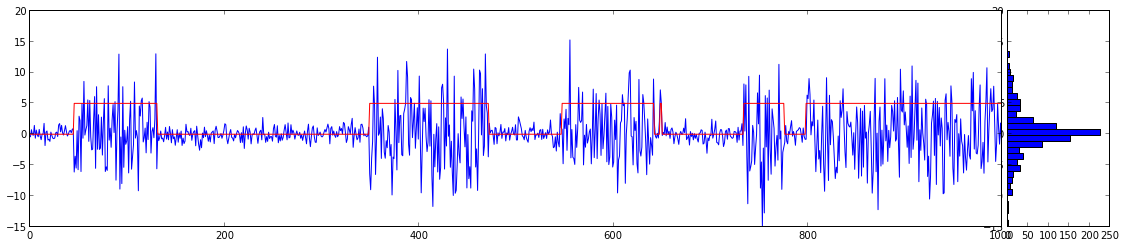Heinrich Hartmann

# Generative Models For Time Series

Written on 2014-12-11

In this note we explore several mechanisms of how to construct time series. For us a time series is a sequence of real numbers

where $t$ is the time index parameter. A model for a time series is a sequence of random variables

Given a time series model we can generate many different time series (instances) by drawing random samples $Y_t(\omega)$ for $\omega \in \Omega$.

The following classes of time series will be discussed:

• IID-Noise processes for various distributions
• Random Walks and difference processes
• Markov Models
• Latent Markov Models
• State Space Models

As we will see this constructions allow us to create a great variety of different time series. The generation of sample time series is the first step in gaining an understanding of these models. Time series analysis is concerned with reversing these constructions and infere model type and parameters from time series data.

## Realization in Python

The easiest representation of a time series is using a simple array:

y = [1,2,1,0,-3,-1]


Elements can be addressed with the syntax y[t]. The drawback of these representation is that there is an explicit length of the time series, which was supposed to go on forever. Also the time dependence is very explicit. It is often not meaningful to say, give me the sample at time $0$, but instead we have a sample $y_t$ at a time $t=now$, and we want to have the next 5 samples, say.

I turns out that, python comes with a built in iterator type that is well suited for representing time series. Iterators are simple objects I that provide a method I.next() that returns the next value of the series. Note that there is no way to access previously generated values.

Iterator object can be conveniently generated using the yield keyword. The following example constructs a white noise iterator:

0.963354997711 0.175658838635 0.995743134001


For convenience we provide a class Series which subclasses the iterator class and implements methods for infix operators, e.g. __add__. In this way we can write Z=Y + 4 for the time series with Z.next() = Y.next() + 4.

Also we have written some plotting helper function

• Plot(S) plots a time series (iterator)
• FancyPlot(S) plots a time series together with the histogram

The source code for these extensions can be found on GitHub.

# Fundamental Examples of Time Series Models

We start by constucting some fundamental examples of time series.

The constant series, $Const(c)$ with value $c \in \mathbb{R}$ is defined as a series with $Y_t = c$.

Given a probability distribution $\mathcal{D}$ on the real line, we say that a process $Y_t$ is $\mathcal{D}$-iid noise if

and all random variables $Y_t$ are independent. The constant series is IID noise with distribution $\delta_c$.

Standard white noise $\epsilon$ is defined as $IID(\mathcal{N}(0,1))$ noise with standard gaussian normal distribution.

Other important distributions for our purposes are the Bernoulli Distribution $\mathcal{D} = Ber_p$, with

and the Binomial Distribution $\mathcal{B}(n,p)$ with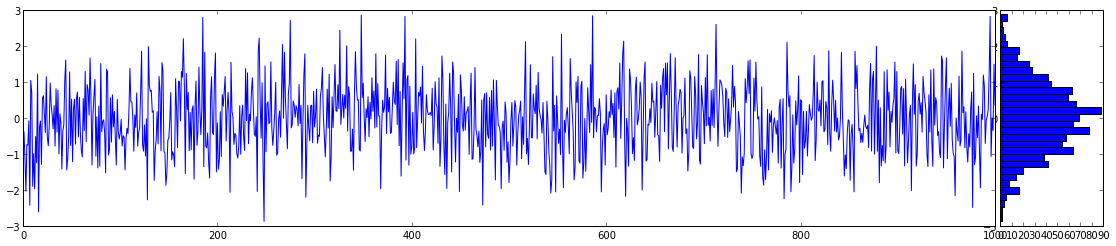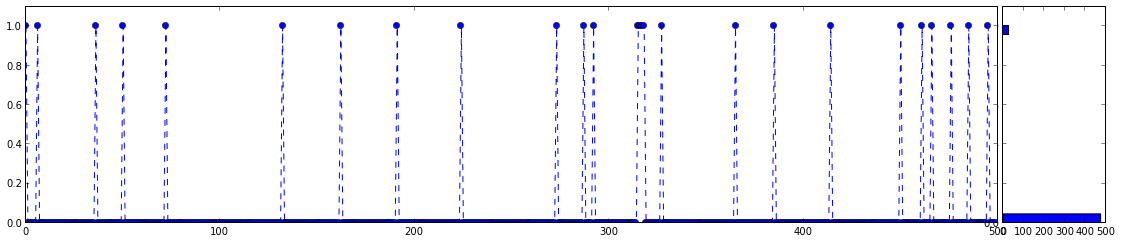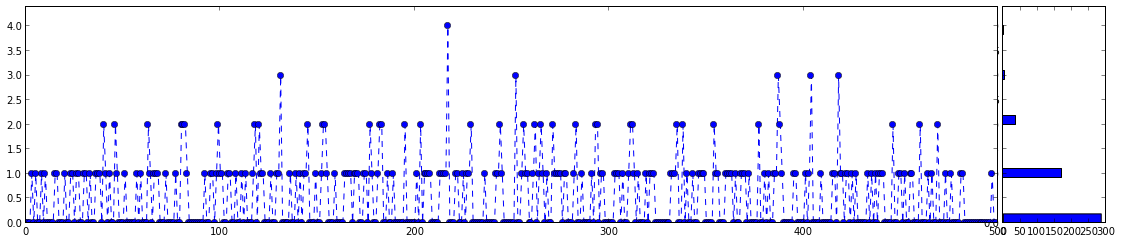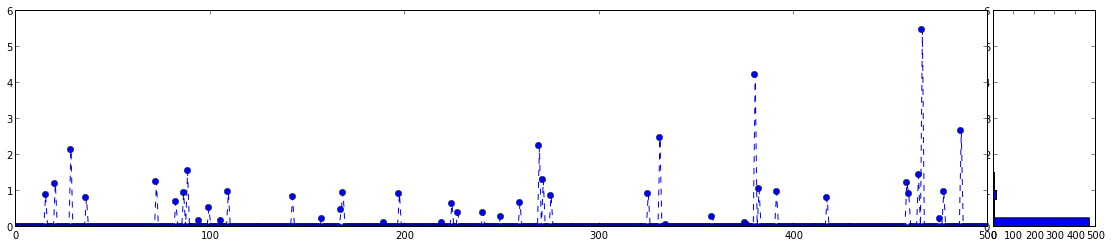# Arithmetic with time series

Our convenience class Series, allows us to do simple arithemtic on time series using the familiar infix notation.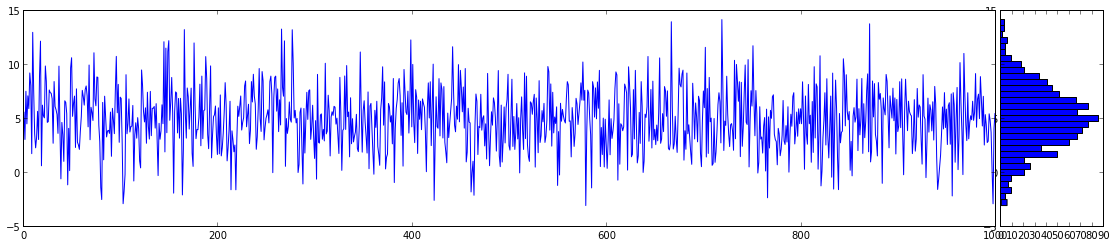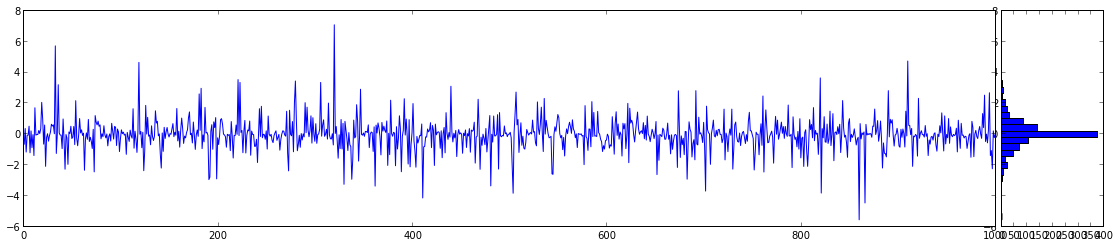# Integration of Series and Random Walks

Random wals are realized as comulative sums over a series.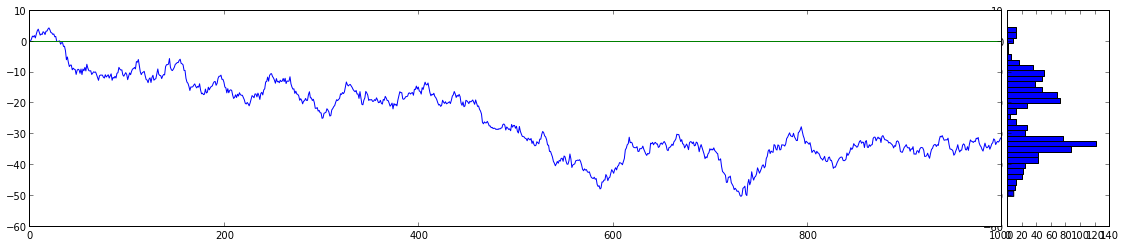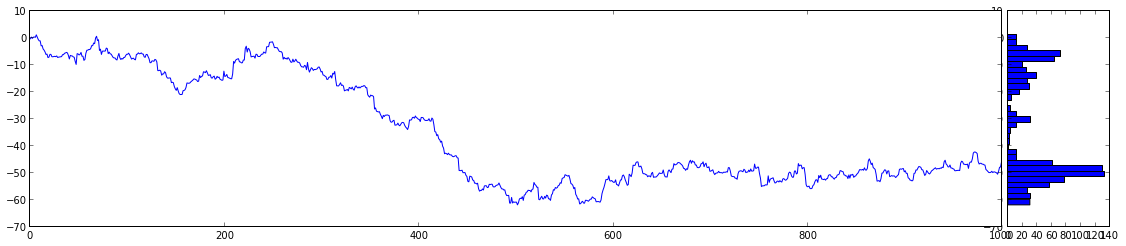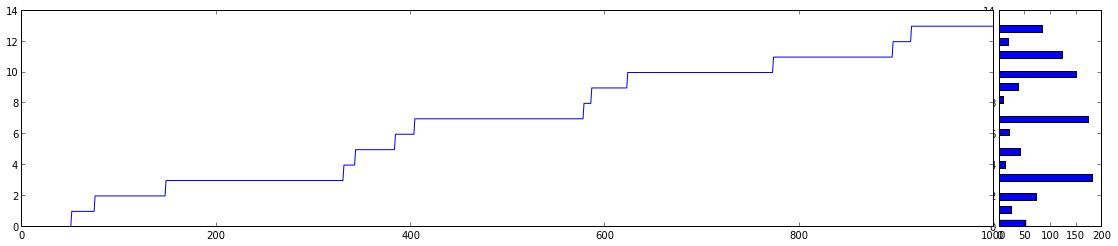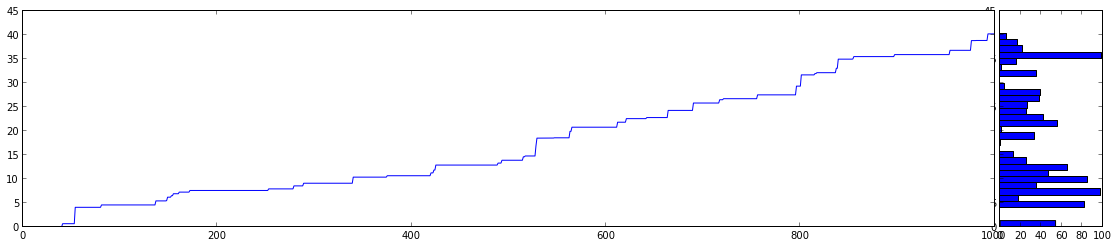# Differencing Time Series

Differencing is the inverse to comulative sums.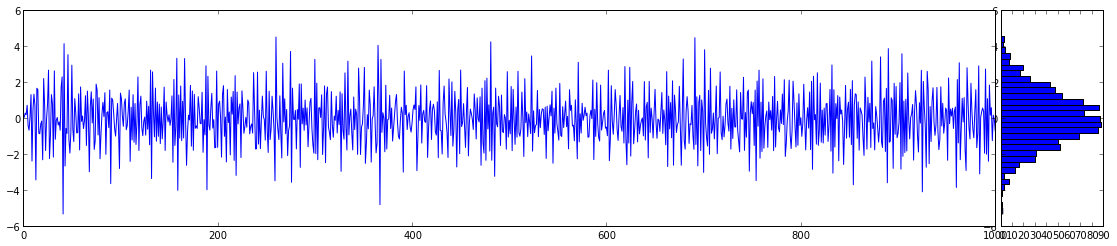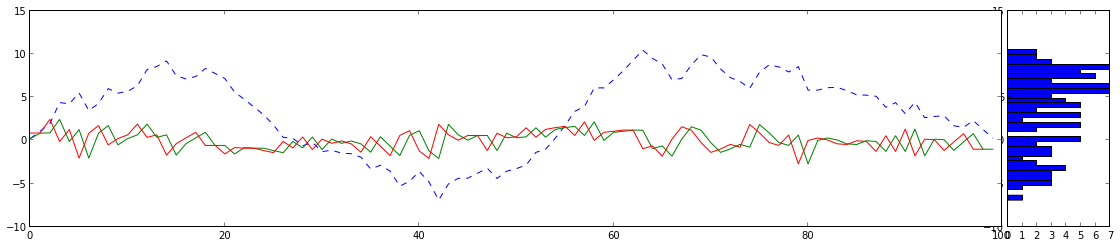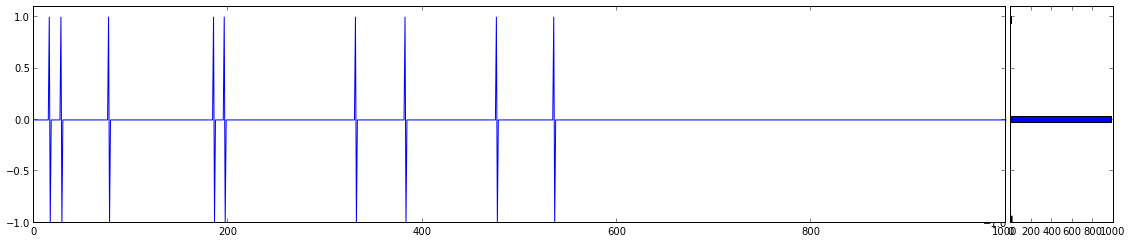# Smoothing and Moving Averages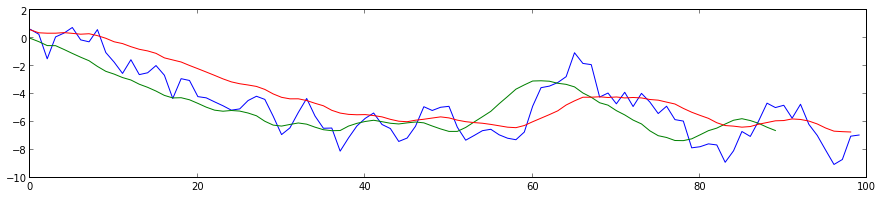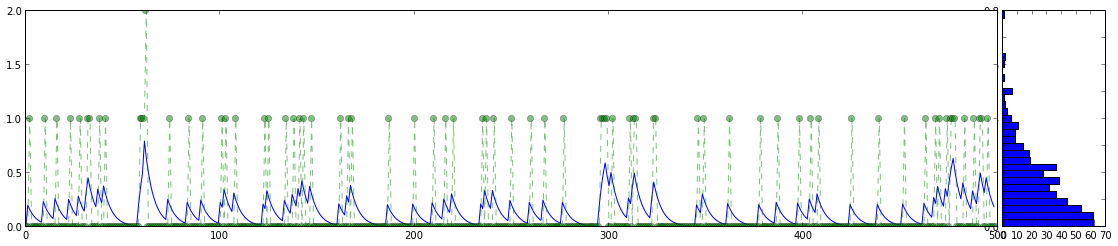# Markov Models

A markov chain consists of

1. a set of states $S$ which we will assume to be natural numbers $S={ 1,2,3,…,N }$,
2. a probability transition matrix $T_{i,j} \in [0,1]$, where $i,j \in S$
3. an initial distribution $\pi$, over $S$.

A realization of a markov chain is a sequence of random variables $Y_t \in S$, such that:

1. The distribution of $Y_0$ is equal to $\pi$.
2. The transition probabilities are given by:

3. The markov property has to hold:

Makrov chains are very easy to generate. We start with a random choice of start state $y_0 \in S$ sampled according to $\pi$. Then, we iteratively generate the further states $y_t$. If we are in a state $s=y_t$ the next state $y_{t+1}$ is ranomly sampled from $1, \dots, N$ according to the the distribution $(T\_{s,1}, \dots, T\_{s,N})$.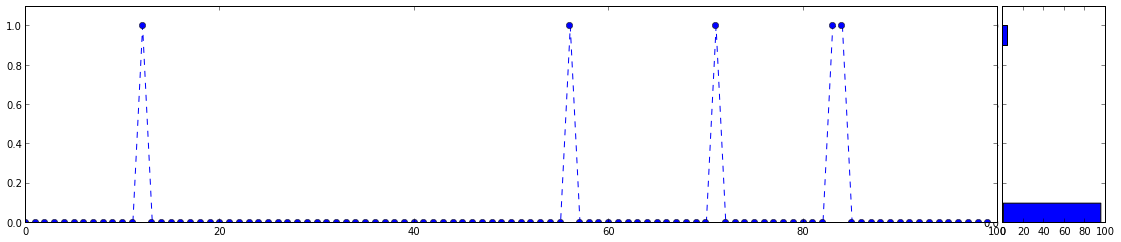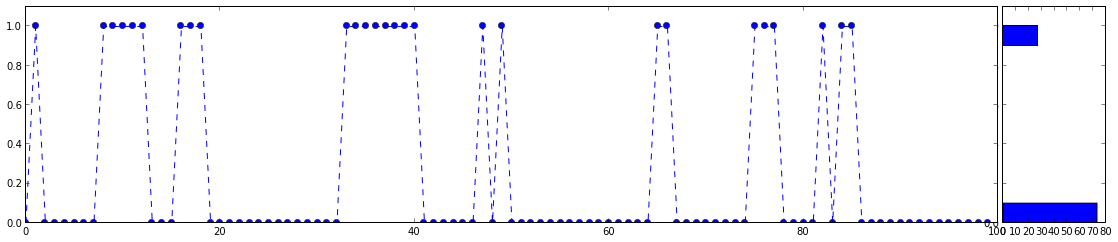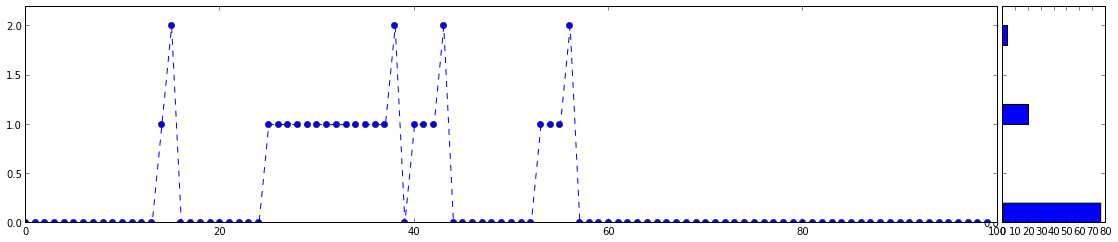# Two-Step Modles

Given a time series model $S_t$ with discrete values in ${1, \dots, K}$, and for each $k = 1…K$ another time series model $Y^k_t$, we can produce a two step model as follows. Depending on the value $S_t$ we select one of the time series $Y^1_t,\dots,Y^K_t$ to draw the sample from:

### Example: Random Jumps

We have $Y_t ~ \N(0,s)$, and $s = 1$ or $s = 10$ depending on a Bernoulli experiment.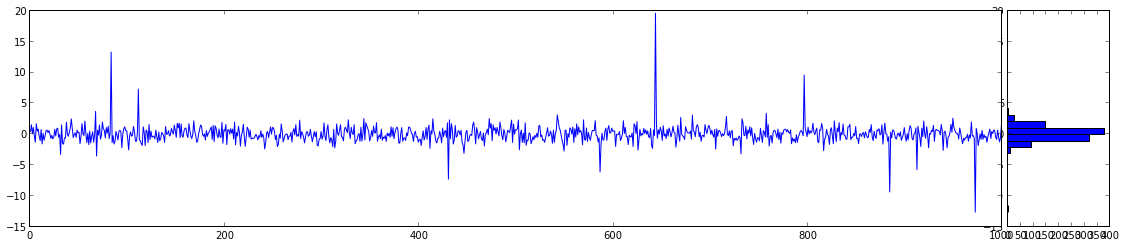### Example: Latent Markov Model

In this case the selector process $S_t$ is a markov model.